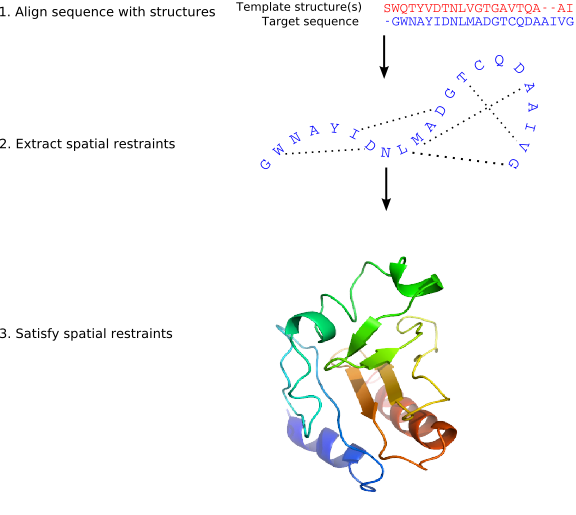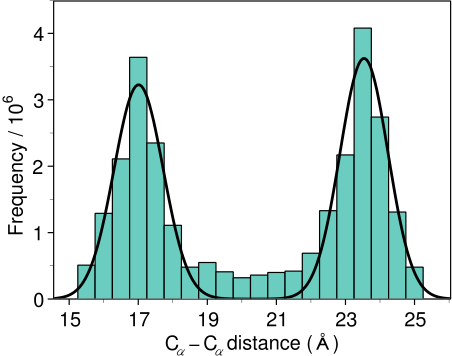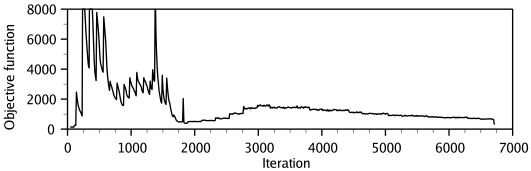# Method for comparative protein structure modeling by MODELLER

MODELLER implements an automated approach to comparative protein structure modeling by satisfaction of spatial restraints (Figure 1.1) [Šali & Blundell, 1993]. The method and its applications to biological problems are described in detail in references listed in Section 1.2. Briefly, the core modeling procedure begins with an alignment of the sequence to be modeled (target) with related known 3D structures (templates). This alignment is usually the input to the program. The output is a 3D model for the target sequence containing all mainchain and sidechain non-hydrogen atoms. Given an alignment, the model is obtained without any user intervention. First, many distance and dihedral angle restraints on the target sequence are calculated from its alignment with template 3D structures (Figure 1.2). The form of these restraints was obtained from a statistical analysis of the relationships between many pairs of homologous structures. This analysis relied on a database of 105 family alignments that included 416 proteins with known 3D structure [Šali & Overington, 1994]. By scanning the database, tables quantifying various correlations were obtained, such as the correlations between two equivalent Cα - Cα distances, or between equivalent mainchain dihedral angles from two related proteins. These relationships were expressed as conditional probability density functions (pdf's) and can be used directly as spatial restraints. For example, probabilities for different values of the mainchain dihedral angles are calculated from the type of a residue considered, from mainchain conformation of an equivalent residue, and from sequence similarity between the two proteins. Another example is the pdf for a certain Cα - Cα distance given equivalent distances in two related protein structures (Figure 1.2). An important feature of the method is that the spatial restraints are obtained empirically, from a database of protein structure alignments. Next, the spatial restraints and CHARMM energy terms enforcing proper stereochemistry [MacKerell et al., 1998] are combined into an objective function. Finally, the model is obtained by optimizing the objective function in Cartesian space. The optimization is carried out by the use of the variable target function method [Braun & Gõ, 1985] employing methods of conjugate gradients and molecular dynamics with simulated annealing (Figure 1.3). Several slightly different models can be calculated by varying the initial structure. The variability among these models can be used to estimate the errors in the corresponding regions of the fold.

There are additional specialized modeling protocols, such as that for the modeling of loops (Section 2.3).Automatic builds 2019-06-19